Question

# Calculation of individual costs and WACC Dillon Labs has asked its financial manager to measure the...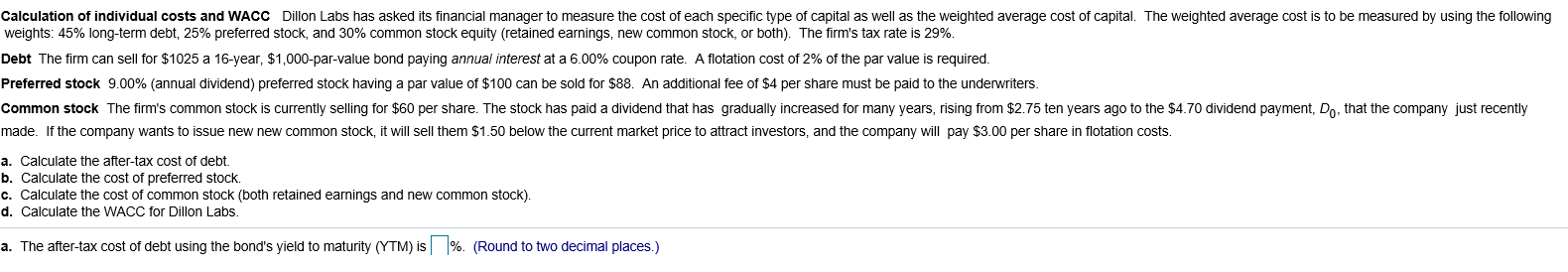Calculation of individual costs and WACC Dillon Labs has asked its financial manager to measure the cost of each specific type of capital as well as the weighted average cost of capital. The weighted average cost is to be measured by using the following weights: 45% long-term debt, 25% preferred stock, and 30% common stock equity (retained earnings, new common stock, or both). The firm's tax rate is 29%. Debt The firm can sell for \$1025 a 16-year, \$1,000-par-value bond paying annual interest at a 6.00% coupon rate. A flotation cost of 2% of the par value is required. Preferred stock 9.00% (annual dividend) preferred stock having a par value of \$100 can be sold for \$88. An additional fee of \$4 per share must be paid to the underwriters. Common stock The firm's common stock is currently selling for \$60 per share. The stock has paid a dividend that has gradually increased for many years, rising from \$2.75 ten years ago to the \$4.70 dividend payment, Do, that the company just recently made. If the company wants to issue new new common stock, it will sell them \$1.50 below the current market price to attract investors, and the company will pay \$3.00 per share in flotation costs. a. Calculate the after-tax cost of debt. b. Calculate the cost of preferred stock. c. Calculate the cost of common stock (both retained earnings and new common stock). d. Calculate the WACC for Dillon Labs. a. The after-tax cost of debt using the bond's yield to maturity (YTM) is %. (Round to two decimal places.)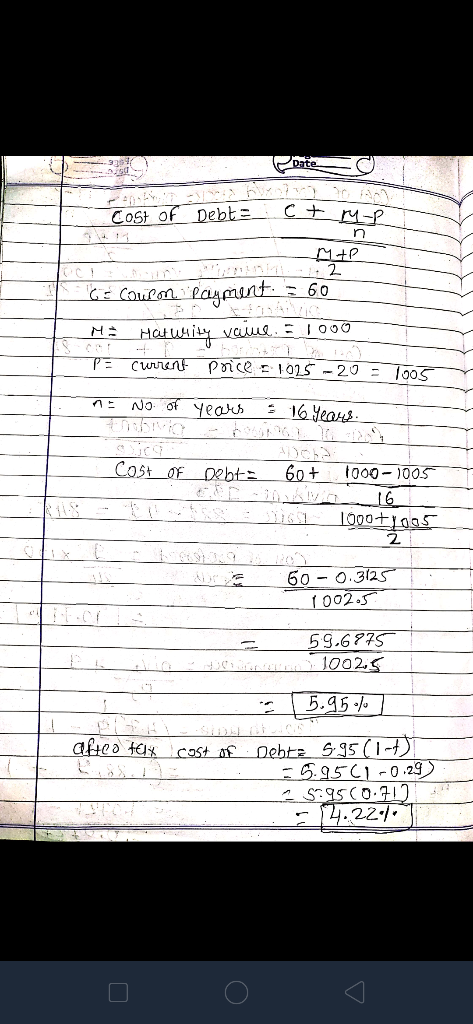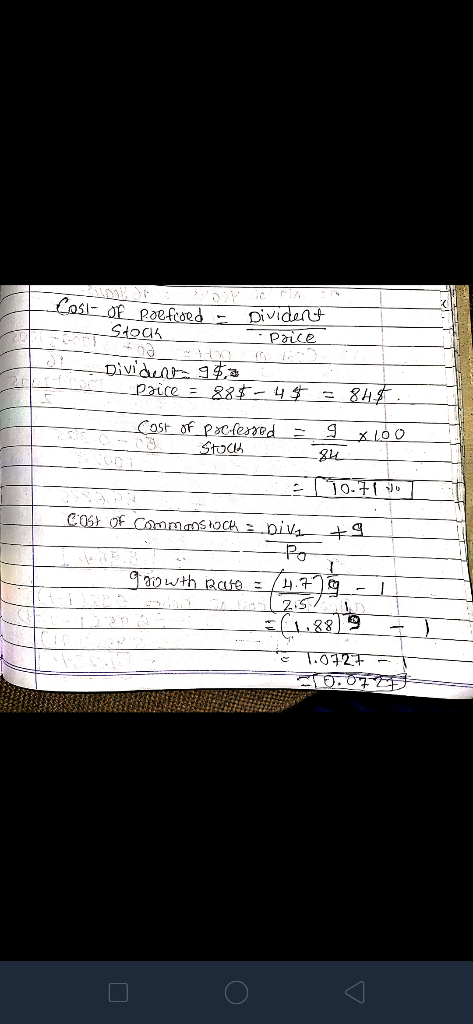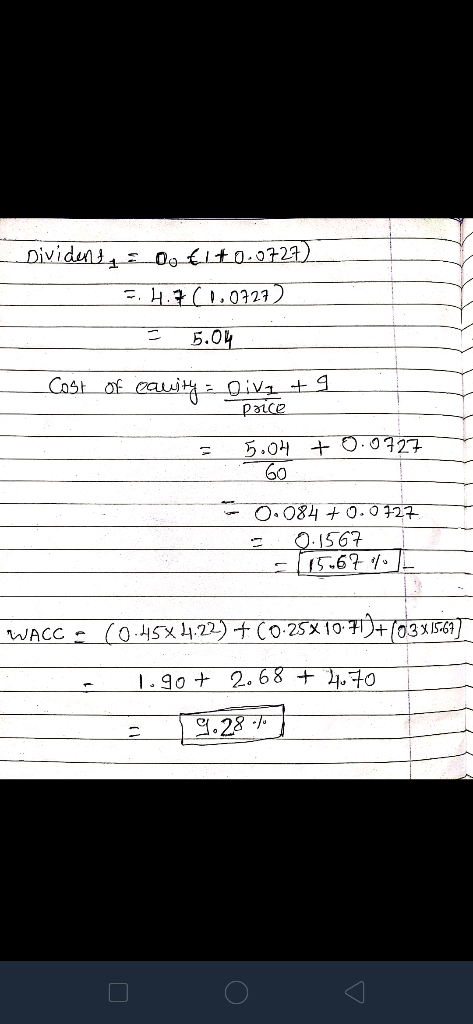I hope my efforts will be fruitful to you...?

#### Earn Coins

Coins can be redeemed for fabulous gifts.

Similar Homework Help Questions
• ### Calculation of individual costs and WACC Dillon Labs has asked its financial manager to measure the...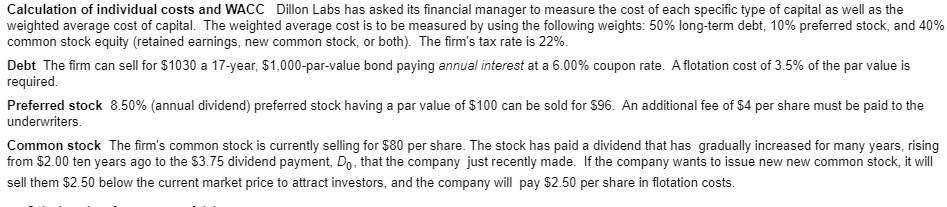Calculation of individual costs and WACC Dillon Labs has asked its financial manager to measure the cost of each specific type of capital as well as the weighted average cost of capital. The weighted average cost is to be measured by using the following weights: 50% long-term debt, 10% preferred stock, and 40% common stock equity (retained earnings, new common stock, or both). The firm's tax rate is 22%. Debt The firm can sell for \$1030 a 17-year, \$1,000-par-value bond...

• ### Calculation of individual costs and WACC Dillon Labs has asked its financial manager to measure the...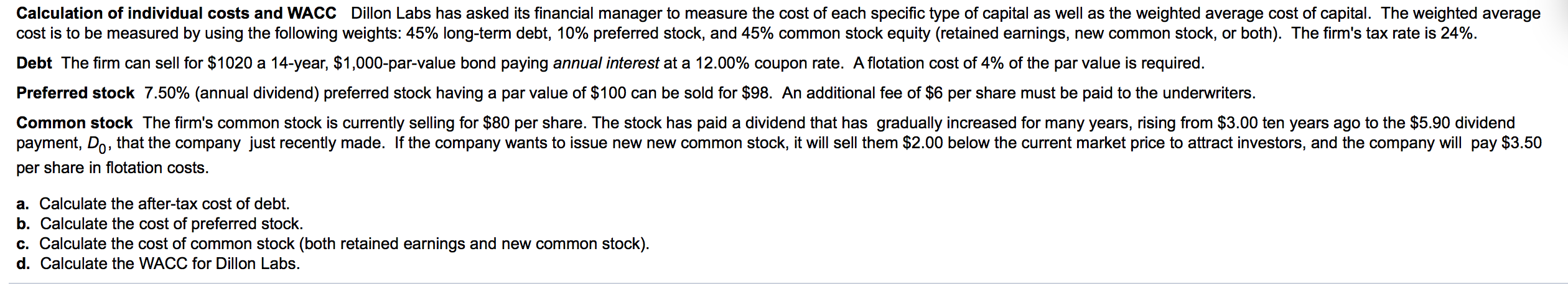Calculation of individual costs and WACC Dillon Labs has asked its financial manager to measure the cost of each specific type of capital as well as the weighted average cost of capital. The weighted average cost is to be measured by using the following weights: 45% long-term debt, 10% preferred stock, and 45% common stock equity (retained earnings, new common stock, or both). The firm's tax rate is 24% Debt The firm can sell for \$1020 a 14-year, \$1,000-par-value bond...

• ### Calculation of individual costs and WACC - Dillon Labs has asked its financial manager to measure...

Calculation of individual costs and WACC - Dillon Labs has asked its financial manager to measure the cost of each specific type of capital as well as the common stock equity (retained earnings, new common stock, or both). The firm's tax rate is 29%. Debt - the firm can sell for \$1010 a 15-year, \$1000-par value bond paying annual interest at a 6.00% coupon rate. A flotation cost of 3.5% of the par value is required. Preferred stock - 9.50%...

• ### Calculation of individual costs and WACC Dillon Labs has asked its financial manager to measure the...

Calculation of individual costs and WACC Dillon Labs has asked its financial manager to measure the cost of each specific type of capital as well as the weighted average cost of capital. The weighted average cost is to be measured by using the following weights: 40% long-term debt, 25% preferred stock, and 35% common stock equity (retained earnings, new common stock, or both). The firm's tax rate is 26%.Debt The firm can sell for \$1030 a 14-year, \$1,000-par-value bond paying...

• ### Calculation of individual costs and WACC   Dillon Labs has asked its financial manager to measure the...

Calculation of individual costs and WACC   Dillon Labs has asked its financial manager to measure the cost of each specific type of capital as well as the weighted average cost of capital. The weighted average cost is to be measured by using the following​ weights:35​%Long-term debt, 15​% preferred​ stock, and 50​% common stock equity​ (retained earnings, new common​ stock, or​ both). The​ firm's tax rate is 22​%. .Debt The firm can sell for ​\$1020 a 16​-year, ​\$1,0001,000​-par-value bond paying annual...

• ### Calculation of individual costs and WACC Dillon Labs has asked its financial manager to measure the...

Calculation of individual costs and WACC Dillon Labs has asked its financial manager to measure the cost of each specific type of capital as well as the weighted average cost of capital. The weighted average cost is to be measured by using the following​ weights: 40​% long-term debt, 10​% preferred​ stock, and 50​% common stock equity​ (retained earnings, new common​ stock, or​ both). The​ firm's tax rate is 28​%. Debt The firm can sell for \$1005 a 15-year, ​\$1,000​-par-value bond...

• ### P9-17 (similar to) Calculation of individual costs and WACC Dillon Labs has asked its financial manager...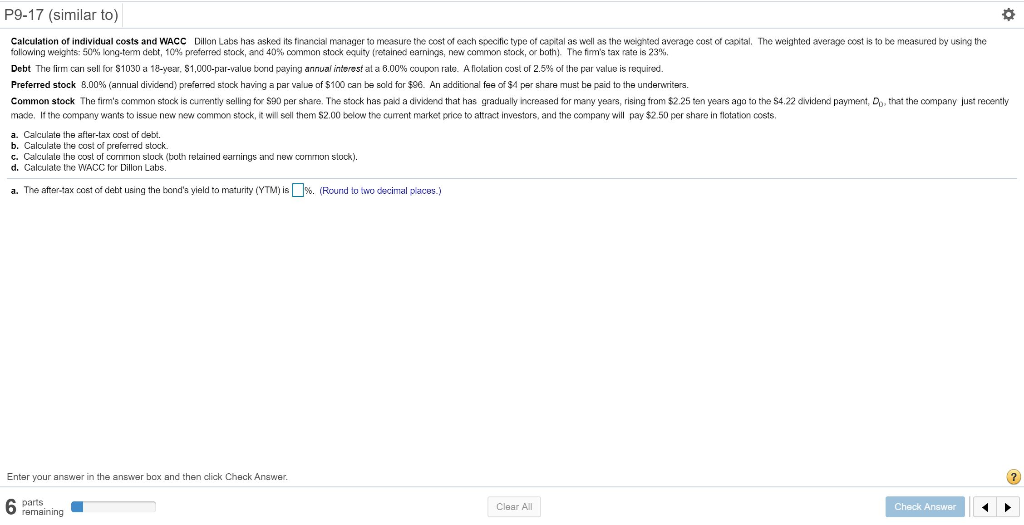P9-17 (similar to) Calculation of individual costs and WACC Dillon Labs has asked its financial manager to measure the cost of each specific type of capital as well as the weighted average cost of capital. The weighted average cost is to be measured by using the following weights: 50% long-term debt, 10% preferred stock, and 40%, common stock equity (retained earnings, new common stock, or both). The firm's tax rate is 23%. Debt The firm can sell for \$1030 a...

• ### P9-17 (similar to) Question Help Calculation of individual costs and WACC Dillon Labs has asked its...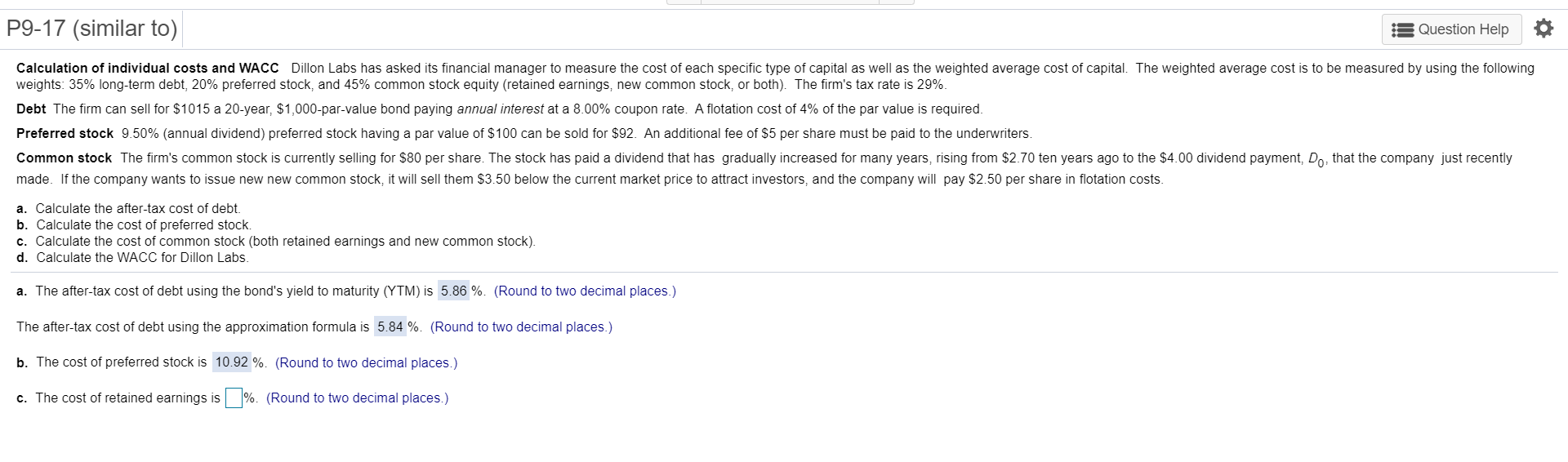P9-17 (similar to) Question Help Calculation of individual costs and WACC Dillon Labs has asked its financial manager to measure the cost of each specific type of capital as well as the weighted average cost of capital. The weighted average cost is to be measured by using the following weights: 35% long-term debt, 20% preferred stock, and 45% common stock equity (retained earnings, new common stock, or both). The firm's tax rate is 29%. Debt The firm can sell for...

• ### Dillon Labs has asked its financial manager to measure the cost of each specific type of...

Dillon Labs has asked its financial manager to measure the cost of each specific type of capital as well as the weighted average cost of capital. The weighted average cost is to be measured by using the following​ weights: 50 % ​long-term debt, 25 % preferred​ stock, and 25 % common stock equity​ (retained earnings, new common​ stock, or​ both). The​ firm's tax rate is 23​%. Debt: The firm can sell for ​\$1010 a 16 ​-year, ​\$1,000 ​-par-value bond paying...

• ### Dillon Labs has asked its financial manager to measure the cost of each specific type of...

Dillon Labs has asked its financial manager to measure the cost of each specific type of capital as well as the weighted average cost of capital. The weighted average cost is to be measured by using the following​ weights: 40​% ​long-term debt, 25​% preferred​ stock, and 35​% common stock equity​ (retained earnings, new common​ stock, or​ both). The​ firm's tax rate is 25​%. Debt The firm can sell for ​\$1010 a 19​-year, ​\$1 comma 000​-par-value bond paying annual interest at...